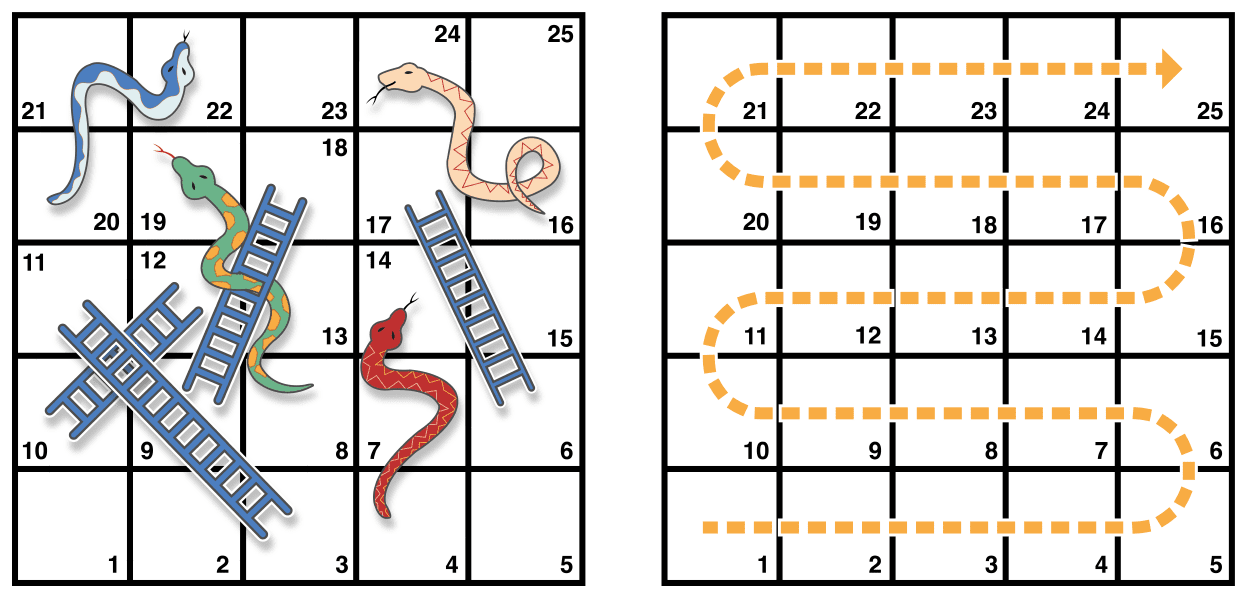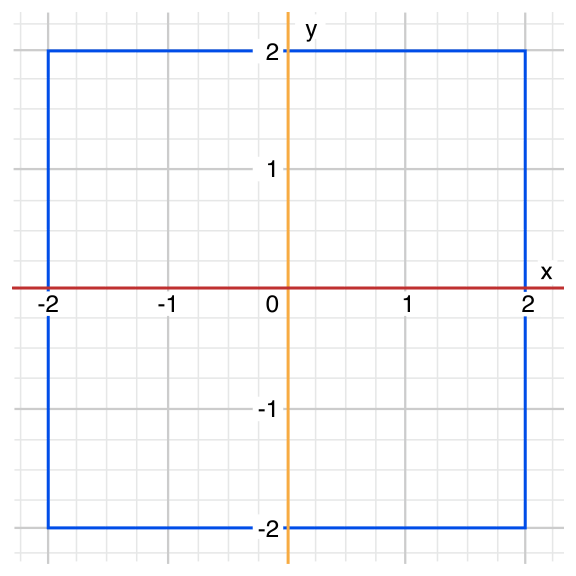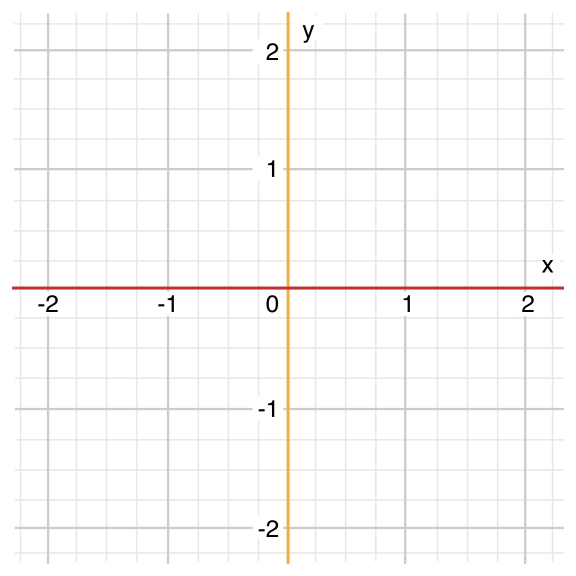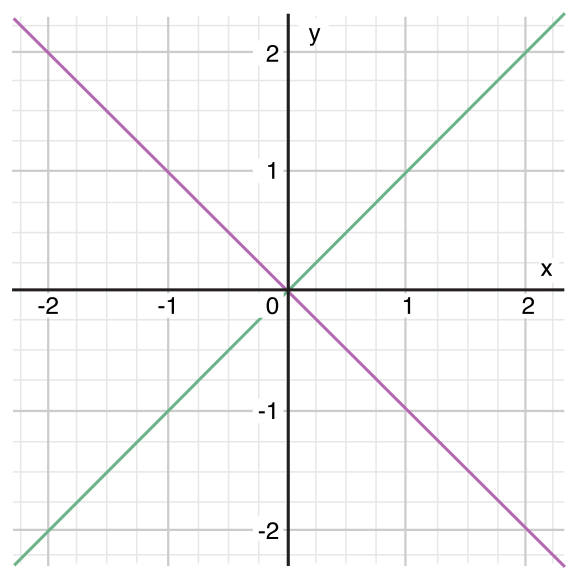# Swift学习之路-控制流

Swift 提供所有多样化的控制流语句。包括 `while` 循环来多次执行任务；` if ，guard ，switch` 语句来基于特定的条件执行不同的代码分支；还有比如 `break``continue` 语句来传递执行流到你代码的另一个点上。

Swift 同样添加了 `for-in` 循环，它让你更简便地遍历数组、字典、范围和其他序列。

Swift 的 `switch` 语句同样比 C 中的对应语句多了不少新功能。比如说 Swift 中的 `switch` 语句不再“贯穿”到下一个情况当中，这就避免了 C 中常见的 `break` 语句丢失问题。情况可以匹配多种模式，包括间隔匹配，元组和特定的类型。 `switch` 中匹配的值还能绑定到临时的常量和变量上供情况中代码使用，并且可以为每一个情况写 `where` 分句表达式来应用复杂条件匹配。

## For-in 循环

``````let names = ["Anna", "Alex", "Brian", "Jack"]
for name in names {
print("Hello, \(name)!")
}
// Hello, Anna!
// Hello, Alex!
// Hello, Brian!
// Hello, Jack!``````

``````let numberOfLegs = ["spider": 8, "ant": 6, "cat": 4]
for (animalName, legCount) in numberOfLegs {
print("\(animalName)s have \(legCount) legs")
}
// ants have 6 legs
// cats have 4 legs
// spiders have 8 legs``````

Dictionary 中的元素没有必要安照它们写入的顺序遍历出来。 Dictionary 的内容内在无序，并且不在取回遍历时保证有序。需要注意的是，你给 Dictionary 插入元素的次序并不能代表你遍历时候的顺序。更多关于数组和字典，见集合类型

for-in 循环同样能遍历数字区间。这个栗子打印了乘五表格的前几行：

``````for index in 1...5 {
print("\(index) times 5 is \(index * 5)")
}
// 1 times 5 is 5
// 2 times 5 is 10
// 3 times 5 is 15
// 4 times 5 is 20
// 5 times 5 is 25``````

``````let base = 3
let power = 10
for _ in 1...power {
}
print("\(base) to the power of \(power) is \(answer)")
// prints "3 to the power of 10 is 59049"``````

``````let minutes = 60
for tickMark in 0..<minutes {
// render the tick mark each minute (60 times)
}``````

``````let minuteInterval = 5
for tickMark in stride(from: 0, to: minutes, by: minuteInterval) {
// render the tick mark every 5 minutes (0, 5, 10, 15 ... 45, 50, 55)
}``````

``````let hours = 12
let hourInterval = 3
for tickMark in stride(from: 3, through: hours, by: hourInterval) {
// render the tick mark every 3 hours (3, 6, 9, 12)
}``````

## While 循环

while 循环执行一个合集的语句直到条件变成 false 。这种循环最好在第一次循环之后还有未知数量的遍历时使用。Swift 提供了两种 while 循环：

• while 在每次循环开始的时候计算它自己的条件；
• repeat-while 在每次循环结束的时候计算它自己的条件。

### While

while 循环通过判断单一的条件开始。如果条件为 true ，语句的合集就会重复执行直到条件变为 false 。

``````while condition {
statements
}``````• 棋盘拥有 25 个方格，目标就是到达或者超过第 25 号方格；
• 每一次，你扔一个六面色子，安装方格的数字移动，依据水平的线路，如图安装上边虚线箭头标注的路线；
• 如果你停留在了梯子的下边，你就可以顺着梯子爬上去；
• 如果你停留在了蛇的头上，你就要顺着蛇滑下来。

``````let finalSquare = 25
var board = [Int](repeating: 0, count: finalSquare + 1)``````

``````board = +08; board = +11; board = +09; board = +02
board = -10; board = -11; board = -02; board = -08``````

``````var square = 0
var diceRoll = 0
while square < finalSquare {
// roll the dice
diceRoll += 1
if diceRoll == 7 { diceRoll = 1 }
// move by the rolled amount
square += diceRoll
if square < board.count {
// if we're still on the board, move up or down for a snake or a ladder
square += board[square]
}
}
print("Game over!")``````

while 循环在这个情况当中合适是因为开始 while 循环之后游戏的长度并不确定。循环会一直执行下去直到特定的条件不满足。

### Repeat-While

while 循环的另一种形式，就是所谓的 repeat-while 循环，在判断循环条件之前执行一次循环代码块。然后会继续重复循环直到条件为 false 。

Swift 的 `repeat-while` 循环是与其他语言中的 `do-while` 循环类似的。

``````repeat {
statements
} while condition``````

``````let finalSquare = 25
var board = [Int](repeating: 0, count: finalSquare + 1)
board = +08; board = +11; board = +09; board = +02
board = -10; board = -11; board = -02; board = -08
var square = 0
var diceRoll = 0``````

``````repeat {
// move up or down for a snake or ladder
square += board[square]
// roll the dice
diceRoll += 1
if diceRoll == 7 { diceRoll = 1 }
// move by the rolled amount
square += diceRoll
} while square < finalSquare
print("Game over!")``````

## 条件语句

Swift 提供了两种方法来给你的代码添加条件分支，就是所谓的 if 语句和 switch 语句。总的来说，你可以使用 if 语句来判定简单的条件，比如少量的可能性。 switch 语句则适合更复杂的条件，比如多个可能的组合，并且在模式匹配的情况下更加有用，可以帮你选择一段合适的代码分支来执行。

### If

``````var temperatureInFahrenheit = 30
if temperatureInFahrenheit <= 32 {
print("It's very cold. Consider wearing a scarf.")
}
// prints "It's very cold. Consider wearing a scarf."``````

if 语句可以提供一个可选语句集，就是所谓的else分句用来在 if 条件为 false 的时候使用。这些语句用 else 关键字明确：

``````temperatureInFahrenheit = 40
if temperatureInFahrenheit <= 32 {
print("It's very cold. Consider wearing a scarf.")
} else {
print("It's not that cold. Wear a t-shirt.")
}
// prints "It's not that cold. Wear a t-shirt."``````

``````temperatureInFahrenheit = 90
if temperatureInFahrenheit <= 32 {
print("It's very cold. Consider wearing a scarf.")
} else if temperatureInFahrenheit >= 86 {
print("It's really warm. Don't forget to wear sunscreen.")
} else {
print("It's not that cold. Wear a t-shirt.")
}
// prints "It's really warm. Don't forget to wear sunscreen."``````

``````temperatureInFahrenheit = 72
if temperatureInFahrenheit <= 32 {
print("It's very cold. Consider wearing a scarf.")
} else if temperatureInFahrenheit >= 86 {
print("It's really warm. Don't forget to wear sunscreen.")
}``````

### Switch

switch 语句会将一个值与多个可能的模式匹配。然后基于第一个成功匹配的模式来执行合适的代码块。 switch 语句代替 if 语句提供了对多个潜在状态的响应。

``````switch some value to consider {
case value 1:
respond to value 1
case value 2,
value 3:
respond to value 2 or 3
default:
otherwise, do something else
}``````

switch 语句一定得使全面。就是说，给定类型里每一个值都得被考虑到并且匹配到一个 switch 情况。如果无法提供一个switch情况给所有可能的值，你可以定义一个默认匹配所有的情况来匹配所有未明确出来的值。这个匹配所有的情况用关键字 default 标记，并且必须在所有情况的最后出现。

``````let someCharacter: Character = "z"
switch someCharacter {
case "a":
print("The first letter of the alphabet")
case "z":
print("The last letter of the alphabet")
default:
print("Some other character")
}
// Prints "The last letter of the alphabet"``````

switch 语句的第一个情况匹配英语字母表里的第一个字母， a ，并且它的第二个情况匹配最后一个字母， z ，由于 switch 必须拥有所有可能的字母的情况，而不是仅仅英语字母表里的字符，这个 switch 语句使用一个 default 情况来匹配所有其他非 a 和 z 的字符。这使得 switch 语句一定是全面的。

#### 没有隐式贯穿

``````let anotherCharacter: Character = "a"
switch anotherCharacter {
case "a":
case "A":
print("The letter A")
default:
print("Not the letter A")
}
// this will report a compile-time error``````

``````let anotherCharacter: Character = "a"
switch anotherCharacter {
case "a", "A":
print("The letter A")
default:
print("Not the letter A")
}
// Prints "The letter A"``````

#### 区间匹配

switch情况的值可以在一个区间中匹配。这个栗子使用了数字区间来为语言中的数字区间进行转换：

``````let approximateCount = 62
let countedThings = "moons orbiting Saturn"
var naturalCount: String
switch approximateCount {
case 0:
naturalCount = "no"
case 1..<5:
naturalCount = "a few"
case 5..<12:
naturalCount = "several"
case 12..<100:
naturalCount = "dozens of"
case 100..<1000:
naturalCount = "hundreds of"
default:
naturalCount = "many"
}
print("There are \(naturalCount) \(countedThings).")
// prints "There are dozens of moons orbiting Saturn."``````

#### 元组

``````let somePoint = (1, 1)
switch somePoint {
case (0, 0):
print("(0, 0) is at the origin")
case (_, 0):
print("(\(somePoint.0), 0) is on the x-axis")
case (0, _):
print("(0, \(somePoint.1)) is on the y-axis")
case (-2...2, -2...2):
print("(\(somePoint.0), \(somePoint.1)) is inside the box")
default:
print("(\(somePoint.0), \(somePoint.1)) is outside of the box")
}
// prints "(1, 1) is inside the box"``````switch 语句决定坐标是否在原点 (0,0) ；在红色的 x 坐标轴；在橘黄色的 y坐标轴；在蓝色的4乘4以原点为中心的方格里；或者在方格外边。

#### 值绑定

switch 情况可以将匹配到的值临时绑定为一个常量或者变量，来给情况的函数体使用。这就是所谓的值绑定因为值是在情况的函数体里“绑定”到临时的常量或者变量的。

``````let anotherPoint = (2, 0)
switch anotherPoint {
case (let x, 0):
print("on the x-axis with an x value of \(x)")
case (0, let y):
print("on the y-axis with a y value of \(y)")
case let (x, y):
print("somewhere else at (\(x), \(y))")
}
// prints "on the x-axis with an x value of 2"``````switch 语句决定坐标是否在在红色的x坐标轴，在橘黄色的y坐标轴；还是其他地方；或不在坐标轴上。

#### Where

switch 情况可以使用 where 分句来检查额外的情况。

``````let yetAnotherPoint = (1, -1)
switch yetAnotherPoint {
case let (x, y) where x == y:
print("(\(x), \(y)) is on the line x == y")
case let (x, y) where x == -y:
print("(\(x), \(y)) is on the line x == -y")
case let (x, y):
print("(\(x), \(y)) is just some arbitrary point")
}
// prints "(1, -1) is on the line x == -y"``````switch 语句决定坐标在绿色的斜线 `x==y` ，还是在紫色的斜线 `x == -y` ，或者都不是。

#### 复合情况

``````let someCharacter: Character = "e"
switch someCharacter {
case "a", "e", "i", "o", "u":
print("\(someCharacter) is a vowel")
case "b", "c", "d", "f", "g", "h", "j", "k", "l", "m",
"n", "p", "q", "r", "s", "t", "v", "w", "x", "y", "z":
print("\(someCharacter) is a consonant")
default:
print("\(someCharacter) is not a vowel or a consonant")
}
// Prints "e is a vowel"``````

``````let stillAnotherPoint = (9, 0)
switch stillAnotherPoint {
case (let distance, 0), (0, let distance):
print("On an axis, \(distance) from the origin")
default:
print("Not on an axis")
}
// Prints "On an axis, 9 from the origin"``````

## 控制转移语句

• `continue`
• `break`
• `fallthrough`
• `return`
• `throw`

continue , break , 和 fallthrough  语句在下边有详细描述。 return 语句在函数中描述，还有 throw 语句在使用抛出函数传递错误中描述。

### Continue

continue 语句告诉循环停止正在做的事情并且再次从头开始循环的下一次遍历。它是说“我不再继续当前的循环遍历了”而不是离开整个的循环。

``````let puzzleInput = "great minds think alike"
var puzzleOutput = ""
let charactersToRemove: [Character] = ["a", "e", "i", "o", "u", " "]
for character in puzzleInput {
if charactersToRemove.contains(character) {
continue
} else {
puzzleOutput.append(character)
}
}
print(puzzleOutput)
// Prints "grtmndsthnklk"``````

### Break

break 语句会立即结束整个控制流语句。当你想要提前结束 switch 或者循环语句或者其他情况时可以在 switch 语句或者循环语句中使用 break 语句。

#### Switch 语句里的 Break

switch 的情况如果只包含注释的话会导致编译时错误。注释不是语句，并且不会导致 switch 情况被忽略。要使用 break 语句来忽略 switch 情况。

``````let numberSymbol: Character = "三"  // Simplified Chinese for the number 3
var possibleIntegerValue: Int?
switch numberSymbol {
case "1", "١", "一", "๑":
possibleIntegerValue = 1
case "2", "٢", "二", "๒":
possibleIntegerValue = 2
case "3", "٣", "三", "๓":
possibleIntegerValue = 3
case "4", "٤", "四", "๔":
possibleIntegerValue = 4
default:
break
}
if let integerValue = possibleIntegerValue {
print("The integer value of \(numberSymbol) is \(integerValue).")
} else {
print("An integer value could not be found for \(numberSymbol).")
}
// prints "The integer value of 三 is 3."``````

### Fallthrough

Swift 中的 Switch 语句不会从每个情况的末尾贯穿到下一个情况中。相反，整个 switch 语句会在第一个匹配到的情况执行完毕之后就直接结束执行。比较而言，C 你在每一个 switch 情况末尾插入显式的 break 语句来阻止贯穿。避免默认贯穿意味着 Swift 的 switch 语句比 C 更加清晰和可预料，并且因此它们避免了意外执行多个 switch 情况。

``````let integerToDescribe = 5
var description = "The number \(integerToDescribe) is"
switch integerToDescribe {
case 2, 3, 5, 7, 11, 13, 17, 19:
description += " a prime number, and also"
fallthrough
default:
description += " an integer."
}
print(description)
// prints "The number 5 is a prime number, and also an integer."``````

fallthrough 关键字不会为switch情况检查贯穿入情况的条件。 fallthrough 关键字只是使代码执行直接移动到下一个情况（或者 default 情况）的代码块中，就像C的标准 switch 语句行为一样。

### 给语句打标签

``````label name: while condition {
statements
}``````

• 要赢得游戏，你必须精确落在第25格上。`finalSquare``board``square``diceRoll` 的值也用和之前一样的方式来初始化：

``````let finalSquare = 25
var board = [Int](count: finalSquare + 1, repeatedValue: 0)
board = +08; board = +11; board = +09; board = +02
board = -10; board = -11; board = -02; board = -08
var square = 0
var diceRoll = 0``````

while 循环条件是 `while square != finaleSquare` ，用来反映你必须精确地落在第25格上：

``````gameLoop: while square != finalSquare {
diceRoll += 1
if diceRoll == 7 { diceRoll = 1 }
switch square + diceRoll {
case finalSquare:
// diceRoll will move us to the final square, so the game is over
break gameLoop
case let newSquare where newSquare > finalSquare:
// diceRoll will move us beyond the final square, so roll again
continue gameLoop
default:
// this is a valid move, so find out its effect
square += diceRoll
square += board[square]
}
}
print("Game over!")``````

• 如果扔的色子将把玩家移动到最后的方格，游戏就结束。 `break gameLoop` 语句转移控制到 while 循环外的第一行代码上，它会结束游戏。
• 如果扔的色子点数将会把玩家移动超过终的方格，那么移动就是不合法的，玩家就需要再次扔色子。 `continue gameLoop` 语句就会结束当前的 while 循环遍历并且开始下一次循环的遍历。
• 在其他所有的情况中，色子是合法的。玩家根据 `diceRoll` 的方格数前进，并且游戏的逻辑会检查蛇和梯子。然后循环结束，控制返回到 while 条件来决定是否要再次循环。

## 提前退出

guard 语句，类似于 if 语句，基于布尔值表达式来执行语句。使用 guard 语句来要求一个条件必须是真才能执行 guard 之后的语句。与 if 语句不同， guard 语句总是有一个 else 分句—— else 分句里的代码会在条件不为真的时候执行。

``````func greet(person: [String: String]) {
guard let name = person["name"] else {
return
}

print("Hello \(name)!")

guard let location = person["location"] else {
print("I hope the weather is nice near you.")
return
}

print("I hope the weather is nice in \(location).")
}

greet(person: ["name": "John"])
// Prints "Hello John!"
// Prints "I hope the weather is nice near you."
greet(person: ["name": "Jane", "location": "Cupertino"])
// Prints "Hello Jane!"
// Prints "I hope the weather is nice in Cupertino."``````

## 检查API的可用性

Swift 拥有内置的对 API 可用性的检查功能，它能够确保你不会悲剧地使用了对部属目标不可用的 API。

``````if #available(iOS 10, macOS 10.12, *) {
// Use iOS 10 APIs on iOS, and use macOS 10.12 APIs on macOS
} else {
// Fall back to earlier iOS and macOS APIs
}``````

``````if #available(platform name version, ..., *) {
statements to execute if the APIs are available
} else {
fallback statements to execute if the APIs are unavailable
}``````